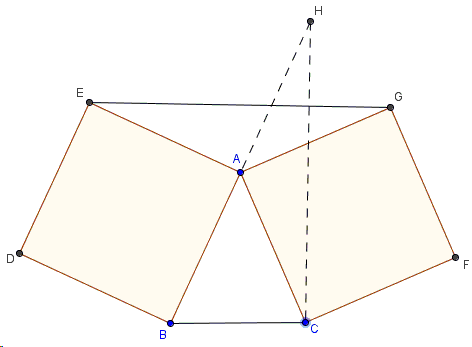# A Degenerate Case of Bottema's Configuration

The applet below illustrates what I shall refer to as Bottema's configuration; the latter consists of two squares $ABDE$ and $ACFG$, and two line segments $BC$ and $EG$:

The specific problem we are concerned with here was offered at the 1988 Leningrad Mathematical Olympiad (9th grade) and included by Ross Honsberger in his Mathematical Diamonds collection:

Squares $ABDE$ and $ACFG$ are drawn outside the triangle $ABC$. Prove that triangle $ABC$ is isosceles if $EG$ is parallel to $BC$.

In 1988, the Soviet 9th graders - close to the USA high school junior year - could be expected to have taken at least one year of probably not very advanced geometry. Thus the original proof (Proof 1) could be said to work from "first principles". Honsberger provides a short but a more sophisticated proof that makes use of the basic geometric transformations - rotation in this case. Finally, there is a one-line solution that exploits an elementary property of Bottema's configuration that is on the borderline of the elementary (meaning "high school") and advanced triangle geometry.

### Proof 1

Assume $AB\ge BC$. Take points $K$ and $L$ on sides $AB$ and $AE$, respectively, so that $AK=AL=AC$. Then draw the angle bisectors $AM$ and $AN$ of $\angle BAC$ and $\angle EAG$, respectively.Since $\angle BAC +\angle EAG=360^{\circ}-\angle BAE-\angle CAG$, we get $\angle BAC+\angle EAG=180^{\circ}$ and $\angle MAK+\angle NAL=90^{\circ}$, $\angle MAN=\angle MAK+\angle NAL+\angle KAL=180^{\circ}$, which implies that $M$, $A$, and $N$ are collinear. Since $AM\perp CK$ and $AN\perp GL$, $CK\parallel GL$. Using the condition $AC\parallel DG$, we obtain that $K=B$, $L=E$, i.e., $\Delta ABC$ is isosceles.

### Proof 2

First of all, Honsberger observes that

With squares around, it's always possible that a quarter-turn or a half-turn might be the key to things. The center of such a rotation is often the center of a square, but this is compromised here by the presence of two squares. However, the squares are joined at the vertex $A$, and a clockwise quarter-turn of $\Delta AEG$ about $A$ is the first step in a beautiful solution.Clearly this rotation takes $G$ to $C$, and since $EG\parallel BC$ to begin with, its image $CH$ is perpendicular to $BC$. Also, $\angle BAH$, being composed of two right angles ($BAE$ and $EAH$), is a straight angle. Furthermore, $AB=AE=AH$. Thus $A$ is the midpoint of the hypotenuse in right triangle $BCH$ and, as its circumcenter, is equidistant from the vertices. Hence, $AB=AC$.

### Proof 3

As a pair, triangles $ABC$ and $AEG$ possess several remarkable properties and are known as flank. In particular, the median from their common vertex $A$ in one is a continuation of the altitude in the other.If, for example, $BM=CM$, making $AM$ a median in $\triangle ABC$, then, as the altitude from A in $\Delta AEG$, $AH\perp EG$. But, since $BC||EG$, $HM$, i.e., $AM$ is also perpendicular to $BC$. Hence, in $\Delta ABC$, $AM$ is both the median and the altitude from $A$, which only possible when $\Delta ABC$ is isosceles.

### References

1. D. Fomin, A. Kirichenko, Leningrad Mathematical Olympiads 1987-1991, MathPro Press, 1994, 80-81
2. R. Honsberger, Mathematical Diamonds, MAA, 2003, 29-30### Bottema's Theorem